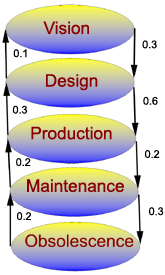## Understanding Business Processes with Coupling From The Past

Coupling From The Past (CFTP) was one of the major advances in probability theory during the 1990s. Now, we see increasing interest in how to leverage its theoretical underpinnings to maximize revenue. The product lifecycle has a particularly straightforward application of statistical principles.

The most fundamental concept in Coupling From The Past is the Markov process. The important properties of a Markov process are

1. At any point in time, the process is in a particular current state
2. After a fixed interval of time, the process changes to its next state
3. The probability of a particular next state depends only on the current state, not on past states

Here is an example business process:There are five possible states: Vision, Design, Production, Maintenance, and Obsolescence. The fixed interval of time is the quarter (i.e., January-March, April-June, July-September, and October-December). The arrows are labeled with the probability of a change to a different state. The process might not change to a different state; in other words, the next state can be the same as the current state. Those probabilities are not shown.

For example, if we are in the Design state, with probability 0.6, the next state is the Production state. With probability 0.1, the next state is the Vision state. Since probabilities sum to 1, with probability 0.3 the next state is the Design state.

We need to find the equilibrium probabilities of these states in order to run our business. If the Vision state has high probability, our business stagnates as we contemplate product ideas with no forward progress. If the Design state has high probability, our time-to-market will be too long, and customers will gravitate toward our competitors' offerings.

We especially need to know the probability of the Production state, since it affects many aspects of resource planning. For example, if the probability of the Production state doubles, we may need to double our available factory floor space and double our number of assembly personnel.

The probability of the Maintenance state has perhaps the most challenging interpretation. Here, we are no longer seeking new markets for the product, but we are still providing customer support, repair, and upgrades. Clearly, this state should not have a negligible probability, since this would drive down customer satisfaction. However, the probability should not be high, since our appeal to investors will decline if too few resources are dedicated to growth.

The Obsolescence state reflects discontinued products. The probability should not be high since this would have a negative impact on branding, and produce a perception of insufficient product focus. A reasonable probability of Obsolescence is desirable, however, since it shows that the management team can make the hard choices to abandon products once they are past their prime.

In Coupling From The Past, we want to find the equilibrium probabilities of the states of a Markov process, but a direct calculation of these probabilities is intractable. In the example business process, the calculation is tractable, and we would not use Coupling From The Past in practice. Our real interest is in any business process that is much more complex and not exactly understood.

## Transition Sets

Although the process is not exactly understood, we are able to generate representative transition sets, and assign those transition sets to a particular time in the past. For example, the transition set for time=-1 is a list of what change would occur if the process were in each state at time=-1. It might be:

• Vision --> Vision
• Design --> Production
• Production --> Design
• Maintenance --> Production
• Obsolescence --> Maintenance

Another of the many possibilities is:

• Vision --> Design
• Design --> Design
• Production --> Production
• Maintenance --> Obsolescence
• Obsolescence --> Obsolescence

In practice, a characteristic of the Markov process is that transitions from one state have some type of formal relationship to transitions from other states. This is known as "Coupling".

When we have a series of consecutive one-step transition sets ending with the transition set for t=-1 to t=0, we can easily determine the multi-step transition sets. We just let the "next" state from t=-n be the "current" state for t=-n+1.

## The Algorithm

Coupling From The Past is an algorithm for finding one equilibrium occurrence of a state. In typical applications, we would run the algorithm thousands or millions of times. The equilibrium probability of a state is the number of equilibrium occurrences of that state divided by the number of runs. For example, we might do 100 runs and find

 State Count Probability Vision 31 .31 Design 10 .10 Production 37 .37 Maintenance 8 .08 Obsolescence 14 .14

Here is the algorithm:

1. Let n=1
2. Choose a single-step transition set from t=-n to t=-n+1
3. Use this to find the multi-step transition set from t=-n to t=0
4. If every "next" state in the multi-step transition set is the same, one equilibrium occurrence of that state has been found, and the algorithm terminates
5. If not, let n=n+1 and go to step 2

Note that step 5 brings us continually backward from t=0. This is the "From The Past" part of the algorithm's name. One important observation is that the maximum value of n is not known in advance. Once we choose a particular single-step transition set, we must keep proceeding through the algorithm until we reach success in step 4. We cannot abandon a run of the algorithm even if n becomes exceedingly large. Coupling From The Past is not for the impatient! The probabilities are correct only if no runs are abandoned.

For more information about Coupling From The Past, see the Web Site for Perfectly Random Sampling with Markov Chains. For more information about probability theory, see Probability in the Open Directory or Probability in the Yahoo! Directory.

Questions about this web site may be sent to web-team@CFTP.COM